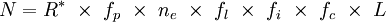from http://en.wikipedia.org/wiki/Drake_equation$drakeequation$

where:

N is the number of civilizations in our galaxy with which we might expect to be able to communicate at any given time

and

R* is the rate of star formation in our galaxy
fp is the fraction of those stars that have planets
ne is average number of planets that can potentially support life per star that has planets
fl is the fraction of the above that actually go on to develop life
fi is the fraction of the above that actually go on to develop intelligent life
fc is the fraction of the above that are willing and able to communicate
L is the expected lifetime of such a civilization
You can use this calculator to easily calculate the effect of changing a variable in the Drake Equation.
 R* fp ne fl fi fc L
You can install this application to your desktop using Adobe Air. Just click below and follow the instructions.